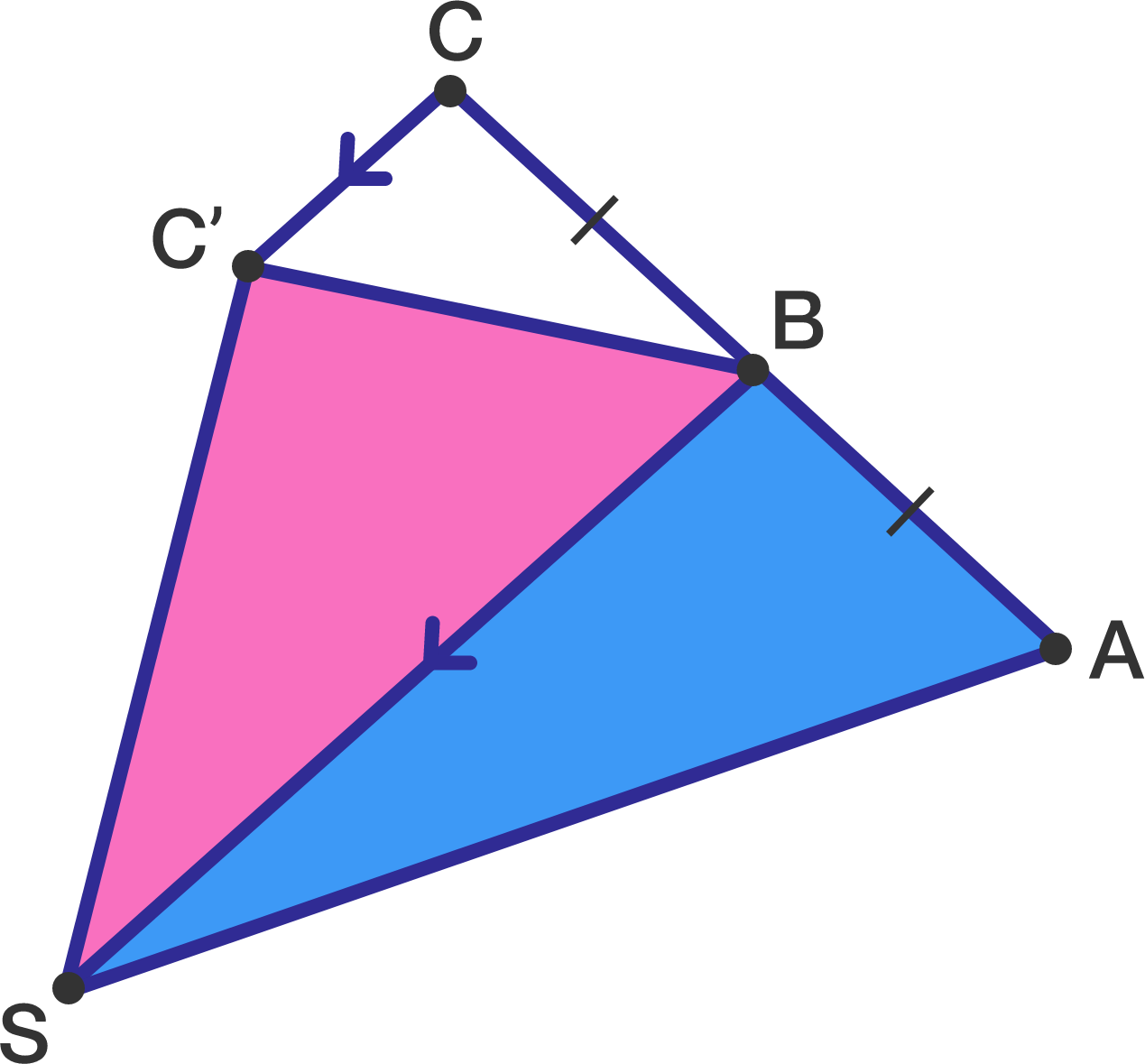# Triangles And Planets

Geometry Level 1

Consider the following picture:• $ABC$ is a straight line
• $CC'$ is parallel to $BS$
• $AB = BC$

If the area of the triangle $ABS$ is 1, what is the area of triangle $BSC'$?

×

Problem Loading...

Note Loading...

Set Loading...